## How to sum largest or smallest 3 values in a list of Excel?

It is common for us to add up a range of numbers by using the SUM function, but sometimes, we need to sum the largest or smallest 3, 10 or n numbers in a range, this may be a complicated task. Today I introduce you some formulas to solve this problem.

Sum the largest / smallest 3 values in a range with formulas

#### Sum the largest / smallest 3 values in a range with formulas

Supposing you have the following range of data need to sum the largest or smallest 3 values.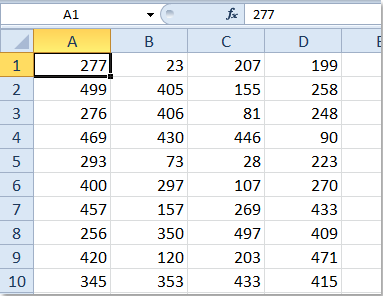Here I can talk about some formulas for you:

1. LARGE function:

You need the LARGE formula three times and then sums the results when you want to sum the top 3 values, do as this:

Enter this LARGE formula into a blank cell =LARGE(A1:D10,1)+ LARGE(A1:D10,2)+ LARGE(A1:D10,3), see screenshot: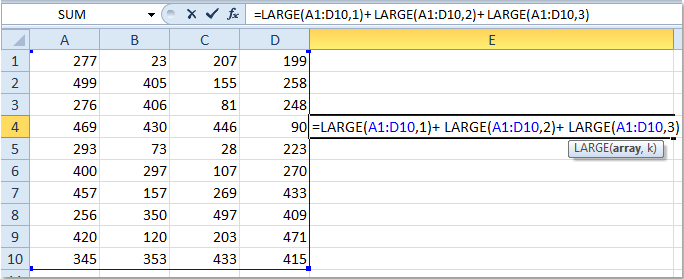And then press Enter key, you will get the result.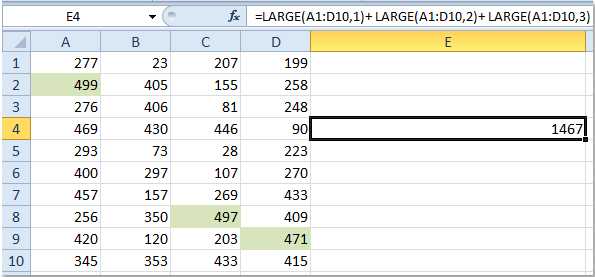Notes:

(1.) If you want to sum the largest 5 values, you need to use it 5 time as this: =LARGE(A1:D10,1)+LARGE(A1:D10,2)+LARGE(A1:D10,3)+LARGE(A1:D10,4)+ LARGE(A1:D10,5) ,you can expand this formula as you need.

(2.) Use the following SMALL function, you can get the result of the smallest 3 values: =SMALL(A1:D10,1)+SMALL(A1:D10,2)+ SMALL(A1:D10,3).

2. Array formulas:

You can also use the following array formulas:

Enter this formula into a blank cell, =SUM(LARGE(A1:D10,{1,2,3})), and then press Ctrl + Shift + Enter keys to get your result .

This formula will become unwieldy as n gets larger. For example, to sum the top 20 values in a range, a formula must contain a list of integers from 1 to 20. Here is a quicker and more convenient array formula: =SUM(LARGE(A1:D10,ROW(INDIRECT ("1:20")))).

After enter this formula, remember to press Ctrl + Shift + Enter keys. If you need to sum a different quantity of numbers, just change the 20 to the desired number.

Note: You can also add up the smallest 3 values with these array formulas as above steps:

=SUM(SMALL(A1:D10,{1,2,3}))

=SUM(SMALL(A1:D10,ROW(INDIRECT ("1:3"))))

#### Sum absolute values in a list with Kutools for Excel

Maybe in some case, you have a list of values which contains positive and negative values, and you just want to sum all the absolute values of them up, and how can you quickly get it done? Kutools for Excel's Sum absolute values function can do a favor for you.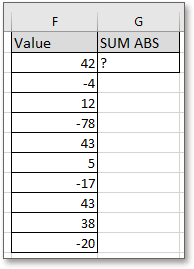After installing Kutools for Excel, please do as below:(

1. Select a blank cell which will place the summed result, and click Kutools > Formula Helper > Formula Helper . See screenshot: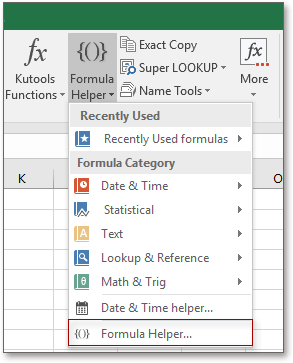2. In the Formula Helper dialog, check Filter checkbox, then type "sum" into the textbox, all formulas about summing are list in the Choose a formula section, click at Sum absolute values, then go to Argument input section to click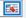to select the list of values you want to sum their absolute value, and then click Ok. See screenshot: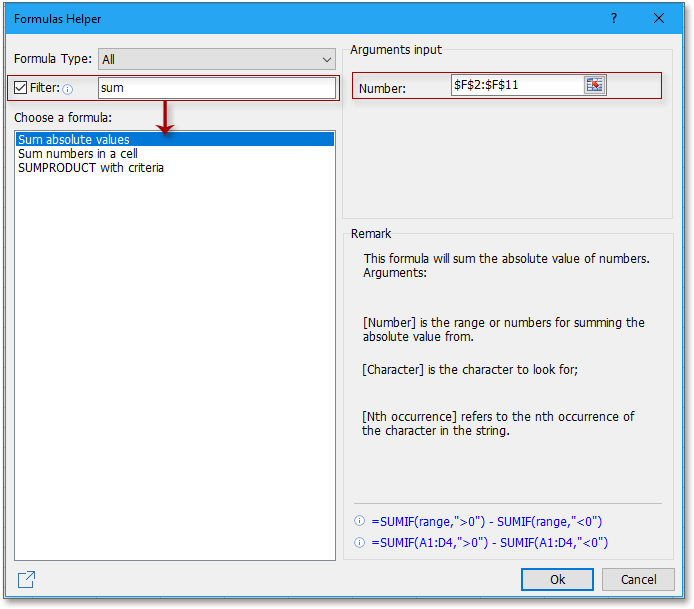Now it has sum up the absolute values of the list right now.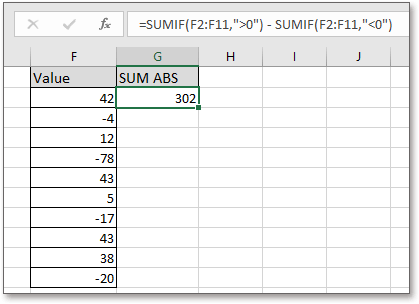### Best Office Productivity Tools

 Popular Features: Find, Highlight or Identify Duplicates   |  Delete Blank Rows   |  Combine Columns or Cells without Losing Data   |   Round without Formula ... Super Lookup: Multiple Criteria VLookup  |   Multiple Value VLookup  |   VLookup Across Multiple Sheets   |   Fuzzy Lookup .... Advanced Drop-down List: Quickly Create Drop Down List   |  Dependent Drop Down List   |  Multi-select Drop Down List .... Column Manager: Add a Specific Number of Columns  |  Move Columns  |  Toggle Visibility Status of Hidden Columns  |  Compare Ranges & Columns ... Featured Features: Grid Focus   |  Design View   |   Big Formula Bar   |  Workbook & Sheet Manager   |  Resource Library (Auto Text)   |  Date Picker   |  Combine Worksheets   |  Encrypt/Decrypt Cells   |  Send Emails by List   |  Super Filter   |   Special Filter (filter bold/italic/strikethrough...) ... Top 15 Toolsets:  12 Text Tools (Add Text, Remove Characters, ...)   |   50+ Chart Types (Gantt Chart, ...)   |   40+ Practical Formulas (Calculate age based on birthday, ...)   |   19 Insertion Tools (Insert QR Code, Insert Picture from Path, ...)   |   12 Conversion Tools (Numbers to Words, Currency Conversion, ...)   |   7 Merge & Split Tools (Advanced Combine Rows, Split Cells, ...)   |   ... and more

Supercharge Your Excel Skills with Kutools for Excel, and Experience Efficiency Like Never Before. Kutools for Excel Offers Over 300 Advanced Features to Boost Productivity and Save Time.  Click Here to Get The Feature You Need The Most...#### Office Tab Brings Tabbed interface to Office, and Make Your Work Much Easier

• Enable tabbed editing and reading in Word, Excel, PowerPoint, Publisher, Access, Visio and Project.
• Open and create multiple documents in new tabs of the same window, rather than in new windows.
• Increases your productivity by 50%, and reduces hundreds of mouse clicks for you every day!No ratings yet. Be the first to rate!
This comment was minimized by the moderator on the site
How to find top values in 3 consecative years out of ten. I have used the =large function but it is only doing best 3 years, not the best 3 consecative years.
This comment was minimized by the moderator on the site
I need to add 4 cells together and subtract 1 cell (biggest number) to get a total.
This comment was minimized by the moderator on the site
Please help me with the formula to sum three of these omitting the lowest and the highest values.: :data1 data2 data3 data4 new data 44.44 26.75 83.80 68.36 65.53
This comment was minimized by the moderator on the site
I need to run a shared work book with appointment's and through code sort them Top 20 largest to Smallest
There are no comments posted here yet